# Chapter 10: Inequalities and limit theorems¶

This Jupyter notebook is the Python equivalent of the R code in section 10.6 R, pp. 447 - 450, Introduction to Probability, 1st Edition, Blitzstein & Hwang.

In :
import matplotlib.pyplot as plt
import numpy as np

%matplotlib inline


## Jensen's inequality¶

Python/NumPy/SciPy make it easy to compare the expectations of $X$ and $g(X)$ for a given choice of $g$, and this allows us to verify some special cases of Jensen's inequality. For example, suppose we simulate 104 times from the $Expo(1)$ distribution:

In :
np.random.seed(24157817)

from scipy.stats import expon

x = expon.rvs(size=10**4)


According to Jensen's inequality, $\mathbb{E}(log \, X) \leq log \, \mathbb{E} \, X$. The former can be approximated by numpy.mean(numpy.log(x)) and the latter can be approximated by numpy.log(numpy.mean(x)), so compute both

In :
meanlog = np.mean(np.log(x))
print('numpy.mean(numpy.log(x)) = {}'.format(meanlog))

logmean = np.log(np.mean(x))
print('numpy.log(numpy.mean(x)) = {}'.format(logmean))

numpy.mean(numpy.log(x)) = -0.5600958563379892
numpy.log(numpy.mean(x)) = 0.00800014338803644


For the $Expo(1)$ distribution, we find that numpy.mean(numpy.log(x)) is approximately −0.56 (the true value is around −0.577), while numpy.log(numpy.mean(x)) is approximately 0 (the true value is 0). This indeed suggests $\mathbb{E}(log \, X) \leq log \, \mathbb{E} \, X$. We could also compare numpy.mean(x**3) to numpy.mean(x)**3, or numpy.mean(numpy.sqrt(x)) to numpy.sqrt(numpy.mean(x)) - the possibilities are endless.

## Visualization of the law of large numbers¶

To plot the running proportion of Heads in a sequence of independent fair coin tosses, we first generate the coin tosses themselves:

In :
np.random.seed(39088169)

from scipy.stats import binom

nsim = 300
p = 1/2
x = binom.rvs(1, p, size=nsim)


Then we compute $\bar{X}_n$ for each value of $n$ and store the results in xbar:

In :
# divide by sequence from 1 to nsim, inclusive
xbar = np.cumsum(x) / np.arange(1, nsim+1)


The above line of code performs element-wise division of the two arrays numpy.cumsum(x) and np.arange(1, nsim+1). Finally, we plot xbar against the number of coin tosses:

In :
x = np.arange(1, nsim+1)
y = xbar

plt.figure(figsize=(12, 4))

plt.plot(x, y, '-', label=r'$\bar{x}$')
plt.hlines(p, 0, nsim,
linestyle='dotted', lw=1.1, alpha=0.5, label=r'$p={}$'.format(p))

plt.xlim([0.0, nsim])
plt.xlabel(r'$n$: number of coin tosses')
plt.yticks([0.0, 0.5, 1.0])
plt.ylabel(r'$\bar{x}_{n}$: proportion of H to $n$')
plt.title('Visualizing the Law of Large Numbers')

plt.legend()

plt.show()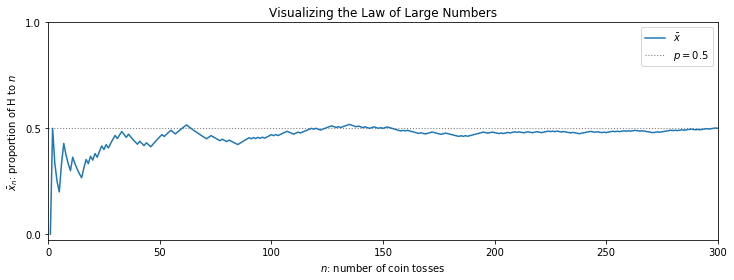You should see that the values of xbar approach p, by the law of large numbers.

## Monte Carlo estimate of $\pi$¶

A famous example of Monte Carlo integration is the Monte Carlo estimate of $\pi$. The unit disk ${(x, y): x^2 +y^2 ≤ 1}$ is inscribed in the square $[−1, 1] \times [−1, 1]$, which has area 4. If we generate a large number of points that are Uniform on the square, the proportion of points falling inside the disk is approximately equal to the ratio of the disk's area to the square’s area, which is $\pi/4$. Thus, to estimate $\pi$ we can take the proportion of points inside the circle and multiply by 4.

In matplotlib.pyplot, to generate Uniform points on the 2D square, we can independently generate the $x$-coordinate and the $y$-coordinate as $Unif(−1, 1)$ r.v.s, using the results of Example 7.1.22:

In :
np.random.seed(63245986)

from scipy.stats import uniform

nsim = 10**6
a = -1
b = 1

x = uniform.rvs(loc=a, scale=b-a, size=nsim)
y = uniform.rvs(loc=a, scale=b-a, size=nsim)


Let's try graphing a small portion of those $x$- and $y$-coordinates.

In :
inside = x**2 + y**2 < 1.0
outside = ~inside

_, ax = plt.subplots(figsize=(8,8))

# we'll graph the first n co-ordinate pairs
# for points inside and points outside of
# x**2 + y**2 = 1.0
n = 5000

ax.plot(x[inside][0:n], y[inside][0:n], '.', color='#fc8d59')
ax.plot(x[outside][0:n], y[outside][0:n], '.', color='#91bfdb')

ax.set_xlim([-1.0, 1.0])
ax.set_xlabel('x')
ax.set_ylim([-1.0, 1.0])
ax.set_ylabel('y')

ax.set_title(r'Monte Carlo estimate of $\pi$: {} / {} points'.format(n, nsim))

plt.show()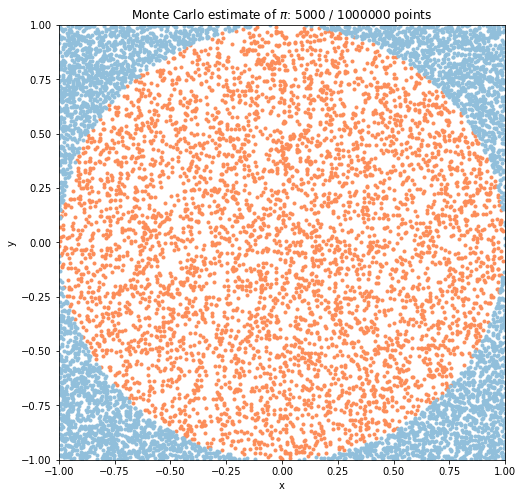To count the number of points in the disk, we use numpy.sum(x**2 + y**2 < 1.0). The array x**2 + y**2 < 1.0 is effectively an indicator vector whose ith element is True (equivalent to 1) if the ith point falls inside the disk, and False (equivalent to 0) otherwise, so the sum of the boolean elements is the number of points in the disk. To get our estimate of $\pi$, we convert the sum into a proportion and multiply by 4. Altogether, we have

In :
num_points_inside = np.sum(x**2 + y**2 < 1.0)
est_pi = 4.0 * num_points_inside / nsim

print('estimated value for pi: {}'.format(est_pi))

estimated value for pi: 3.143172


How close was your estimate to the actual value of $\pi$?

## Visualizations of the central limit theorem¶

One way to visualize the central limit theorem for a distribution of interest is to plot the distribution of $\bar{X}_{n}$ for various values of $n$, as in Figure 10.5. To do this, we first have to generate i.i.d. $X_{1}, \, \ldots, \, X_{n}$ a bunch of times from our distribution of interest. For example, suppose that our distribution of interest is $Unif(0, 1)$, and we are interested in the distribution of $\bar{X}_{12}$, i.e., we set $n = 12$. In the following code, we create a matrix of i.i.d. standard Uniforms. The matrix has 12 columns, corresponding to $X_{1}$ through $X_{12}$. Each row of the matrix is a different realization of $X_{1}$ through $X_{12}$.

In :
np.random.seed(102334155)

nsim = 10**4
n = 12

x = uniform.rvs(size=n*nsim).reshape((nsim, n))

print('matrix x has shape: {}'.format(x.shape))

matrix x has shape: (10000, 12)


Now, to obtain realizations of $\bar{X}_{12}$, we simply take the average of each row of the matrix x; we can do this by calling the numpy.ndarray.mean method on numpy.array object x, specifying axis=1 to take the average of each row of matrix x:

In :
xbar = x.mean(axis=1)


Finally, we create a histogram:

In :
plt.figure(figsize=(10, 4))

plt.hist(xbar, bins=20)

plt.title(r'Histogram of $\bar{X}_{12}$, $X_i \sim Unif(0,1)$')
plt.xlabel(r'$\bar{x}$')
plt.ylabel(r'Frequency')

plt.show()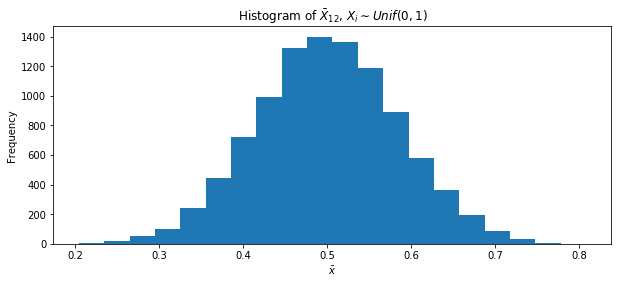You should see a histogram that looks Normal. Because the $Unif(0, 1)$ distribution is symmetric, the CLT kicks in quickly and the Normal approximation for $\bar{X}_{n}$ works quite well, even for $n = 12$. Changing scipy.stats.uniform to scipy.stats.expon, we see that for $X_j$ generated from the $Expo(1)$ distribution, the distribution of $\bar{X}_{n}$ remains skewed when $n = 12$, so a larger value of $n$ is required before the Normal approximation is adequate.

In :
np.random.seed(165580141)

from scipy.stats import expon

n1, n2, n3 = 12, 32, 256

x1 = expon.rvs(size=n1*nsim).reshape((nsim, n1))
x2 = expon.rvs(size=n2*nsim).reshape((nsim, n2))
x3 = expon.rvs(size=n3*nsim).reshape((nsim, n3))

xbar1 = x1.mean(axis=1)
xbar2 = x2.mean(axis=1)
xbar3 = x3.mean(axis=1)

_, (ax1, ax2, ax3) = plt.subplots(1, 3, sharey=True, figsize=(14, 5))

ax1.hist(xbar1, bins=40, color='#1b9e77')
ax1.set_ylabel('Frequency')
ax1.set_title(r'Histogram of $\bar{X}_{12}$, $X_j \sim \, Expo(1)$')

ax2.hist(xbar2, bins=40, color='#d95f02')
ax2.set_title(r'Histogram of $\bar{X}_{32}$, $X_j \sim \, Expo(1)$')

ax3.hist(xbar3, bins=40, color='#7570b3')
ax3.set_title(r'Histogram of $\bar{X}_{256}$, $X_j \sim \, Expo(1)$')

plt.show()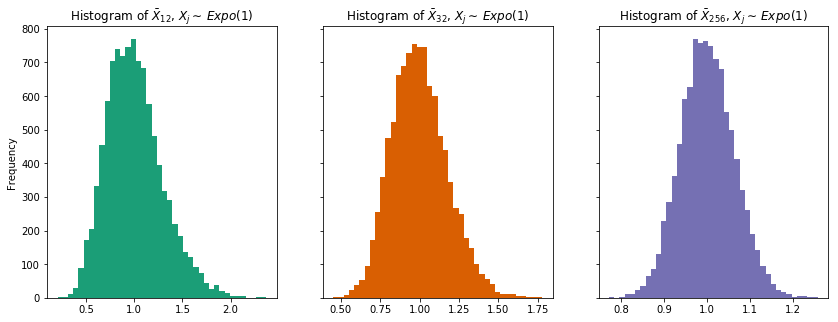Have a look at Appendix A below for another neat visualization of the CLT.

## Chi-Square and Student-$t$ distributions¶

Although the Chi-Square is just a special case of the Gamma (refer to scipy.stats.gamma), it still has its own functions in scipy.stats.chi2: chi2.pdf(x, n) and chi2.cdf(x, n) return the values of the $\chi^2_{n}$ PDF and CDF at x; and chi2.rvs(n, size=nsim) generates nsim $\chi^2_{n}$ r.v.s.

The graph below illustrates Theorem 10.4.2:

In :
from scipy.stats import chi2, gamma

_, (ax1, ax2) = plt.subplots(1, 2, sharey=True, figsize=(14, 6))

x = np.linspace(0, 20, 1000)

n_vals = [1, 2, 4, 8, 16]
alphas = [1.0, .7, .5, .3, .2]

# graph for Chi-Square
for i,n in enumerate(n_vals):
ax1.plot(x, chi2.pdf(x, n), lw=3.2, alpha=alphas[i], color='#33aaff', label='n={}'.format(n))
ax1.set_title(r'$X \sim \chi^2_{n}$')
ax1.set_xlim((0, 20.0))
ax1.set_ylim((0.0, 0.5))
ax1.legend()

# graph for Gamma
lambd = 0.5
for i,n in enumerate(n_vals):
ax2.plot(x, gamma.pdf(x, n/2, scale=1/lambd),
lw=3.2, alpha=alphas[i], color='#ff9933', label='n={}'.format(n))
ax2.set_title(r'$X \sim Gamma(\frac{n}{2}, \frac{1}{2})$')
ax2.set_xlim((0, 20.0))
ax2.set_ylim((0.0, 0.5))
ax2.legend()

plt.suptitle((r'Theorem 10.4.2: $\chi^2_{n}$ distribution is the $Gamma(\frac{n}{2},' r' \, \frac{1}{2})$ distribution'))

plt.show()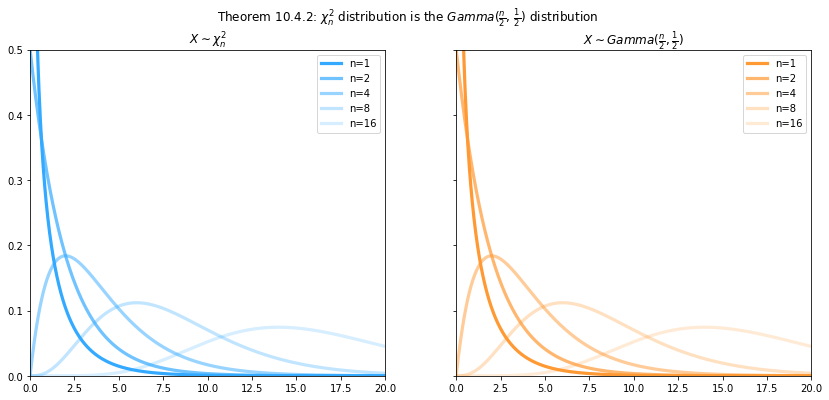The Student-$t$ distribution is supported in scipy.stats.t. To evaluate the PDF or CDF of the $t_n$ distribution at $x$, we use t.pdf(x, n) or t.cdf(x, n). To generate nsim r.v.s from the $t_{n}$ distribution, we use t.rvs(n, size=nsim). Of course, t.pdf(x, 1) is the same as scipy.stats.cauchy's cauchy.pdf(x).

In :
from scipy.stats import t, cauchy

_, (ax1, ax2) = plt.subplots(1, 2, sharey=True, figsize=(14, 6))

x = np.linspace(0, 20, 1000)

# graph for Student-t
y1 = t.pdf(x,1)
ax1.plot(x, y1, lw=3.2, alpha=0.8, color='#33aaff')
ax1.set_title(r'Student-$t$: $\tt{t.pdf(x, 1)}$')

# graph for Cauchy
y2 = cauchy.pdf(x)
ax2.plot(x, y2, lw=3.2, alpha=0.8, color='#ff9933')
ax2.set_title(r'Cauchy: $\tt{cauchy.pdf(x)}$')

plt.suptitle(r'Student-$t$ vs. Cauchy')

plt.show()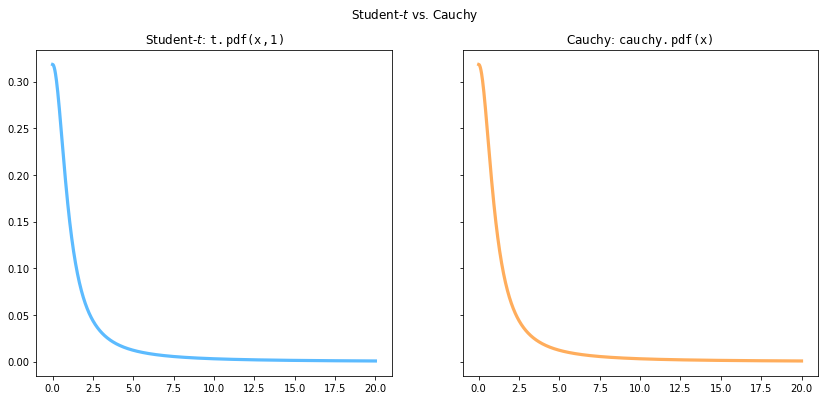## Appendix A: Quincunx - an interactive visualization¶

Statistician and geneticist Francis Galton invented the quincunx, or bean machine, to illustrate the Normal distribution. Here is an implementation built with IPython and D3.js (version 5.7.0), embedded right in this notebook.

In this interactive visualization of the CLR, you can:

• alter the animation by controlling the time between redraws with the delay control (from 50 to 1000 milliseconds, changes are immediately effective without restarting the animation)
• change the number of bins with the num. bins control (from 1 to 25, board is redrawn upon completion of change)
• change the probability $p$ that the ball will go to the right (slider moves left to 0.00, right to 1.00, board is redrawn upon completion of change)
• as the balls drop into the bins below, the heights of the bins will increase to form a histogram
• the running percentage of balls per bin is displayed on each bin and updated in real-time (Math.floor is used, so some accuracy is lost)

First, we import display, Javascript and HTML from the IPython.display module.

Next we use display to load the D3.js file from the Google Hosted Libraries content delivery network. We also load the main JavaScript code in assets/quincunx.js and Cascading Style Sheet definitions in assets/quincunx.html.

Lastly, we embed a small JavaScript snippet into the code cell below to provide an entry point to the quincunx module in assets/quincunx.js.

In :
from IPython.display import display, Javascript, HTML

display(Javascript("""
require.config({
paths: {
}
});
"""))
display(Javascript(filename="./assets/quincunx.js"))
display(HTML(filename="./assets/quincunx.html"))

Javascript("""
(function(element){
require(['quincunx'], function(quincunx) {
quincunx(element.get(0))
});
})(element);
""")

Out:

Can you use the central limit theorem to explain why the distribution of particles at the bottom is approximately Normal?

This interactive visualization extends ideas from the following blogposts:

© Blitzstein, Joseph K.; Hwang, Jessica. Introduction to Probability (Chapman & Hall/CRC Texts in Statistical Science).